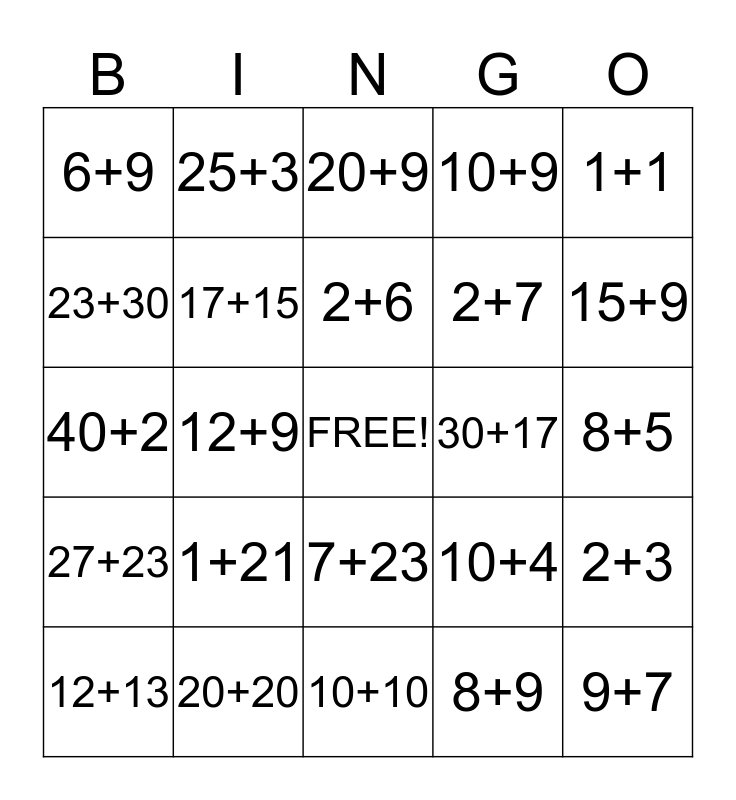This bingo card has a free space and 24 words: 1+21, 20+20, 2+6, 12+13, 1+1, 6+9, 23+30, 25+3, 30+17, 7+23, 2+7, 40+2, 10+4, 2+3, 17+15, 8+9, 8+5, 20+9, 15+9, 12+9, 9+7, 27+23, 10+9 and 10+10.

## Play Online